3rd Grade Science : Use collected data to show patterns in motion

Example Questions

Example Question #21 : Predictions Of Future Motion Through Patterns

Ashley wants to investigate how objects move and see if she can make correct predictions. She knows she will have to observe the object's motion. She wants to see which surface material will allow a toy car to get down a ramp in the least amount of time. She tests the car on wood, plastic, carpet, and grass.

Using the collected data, which answer choice states a pattern that can be identified regarding the car's motion?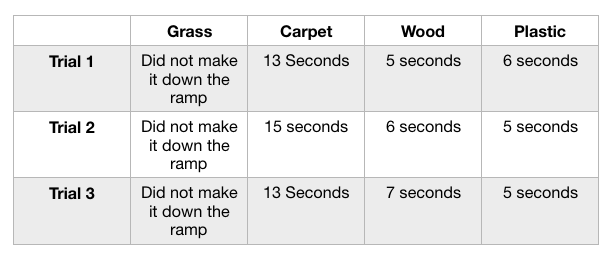The car on the plastic surface went down the ramp fastest two out of three trials.

The car on the plastic surface went down the ramp slowest two out of three trials.

The car on the plastic surface went down the ramp fastest one out of three trials.

The car on the plastic surface went down the ramp slowest of the trials.

The car on the plastic surface went down the ramp fastest two out of three trials.

Explanation:

There is more than one pattern that may emerge from data collected during experiments or investigations. In this investigation, Ashley could see a pattern with the car that rolled down the plastic surface. The car on the plastic ramp rolled down the fastest two out of three trials. Ashley could conclude that this car went down the ramp the fastest.

Example Question #22 : Predictions Of Future Motion Through Patterns

Using the patterns within the data table below, predict the motion of the ball on bounce 4.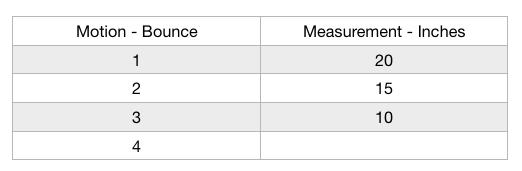7

5

10

0

5

Explanation:

Data collected during experiments or investigations are a great way to look for patterns. In this experiment, there is a bounce (motion) that is measured in inches. Using the trends in the table, we can predict what the next measurement will be. The ball decreases it's bounce by five inches each time, so we can predict that the final bounce will be five inches.

Example Question #23 : Predictions Of Future Motion Through Patterns

A ball is released at the top of a curved ramp, as seen below. Each time the ball reaches the opposite side, it is measured and recorded. Based on the patterns in the data, what would the ball's measurement be on the fifth roll?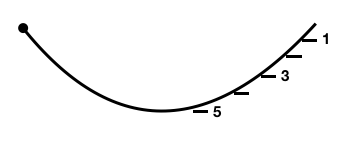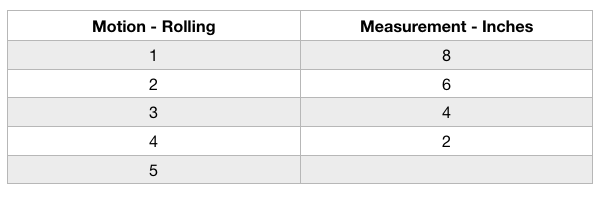3

2

0

1

0

Explanation:

Patterns often emerge in collected data that help us to make predictions about future motion. In this investigation, the measurements are decreasing in two-inch increments. Based on this information, it can be predicted that the final measurement, number five, will be 0 inches. The ball does not gain any additional momentum during its time rolling, only decreasing. The increments are steady so that a pattern can be interpreted for future measurements.

Example Question #24 : Predictions Of Future Motion Through Patterns

Sally and Reina collect data during an experiment and believe that they can use patterns to predict the future motion of objects. Based on the data table below, what can they predict will happen with a marble that weighs 50 grams?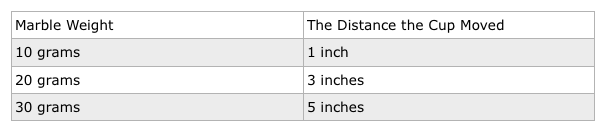The cup will move further than five inches.

The cup will move less than five inches.

There is not enough information to make a prediction.

The cup will move five inches.

The cup will move further than five inches.

Explanation:

This data reveals a pattern about the cup's motion based on the measurements taken. The cup moves a greater distance as the marble's weight increases. An appropriate prediction would be that the cup would move farther than five inches if the marble were to weigh 50 grams.

Example Question #25 : Predictions Of Future Motion Through Patterns

How far would the cup travel if a 50-gram marble were rolled into it based on the patterns in the data table below?9 inches

7 inches

15 inches

5 inches

9 inches

Explanation:

If a 50-gram marble were rolled into the cup, the distance would increase. There is a pattern between the weights of the cup and the movement. Each time the mass of the cup is increased by ten grams, the cup moves two inches. If using the logic created by this pattern, a 50-gram marble would move the cup a total of nine inches. A 40-gram marble would move the cup seven inches (5+2=7), so another ten grams would mean another two inches added (7+2=9) totaling nine grams.

Example Question #26 : Predictions Of Future Motion Through Patterns

Jill wants to investigate how objects move and see if she can make correct predictions. She knows she will have to observe the object's motion. She wants to see how high different bouncy balls will go on the same surface.

If Jill performs the fourth trial, what prediction can be made about "Ball 3"?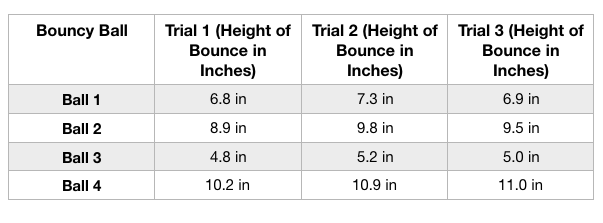"Ball 3" will most likely bounce the highest distance during Trial 4.

"Ball 3" will most likely bounce the shortest distance during Trial 4.

The ball will no longer bounce because it has been used too many times.

There is no way to predict what "Ball 3" will do during Trial 4.

"Ball 3" will most likely bounce the shortest distance during Trial 4.

Explanation:

Jill can make predictions about what will happen in future trials because there are patterns in motion that data helps us to recognize. "Ball 3" bounced the shortest height in all of the previous tests, so, likely, it will again bounce the shortest height. Using patterns and data can help support the prediction that she is making.

Example Question #27 : Predictions Of Future Motion Through Patterns

Ashley wants to investigate how objects move and see if she can make correct predictions. She knows she will have to observe the object's motion. She wants to see which surface material will allow a toy car to get down a ramp in the least amount of time. She tests the car on wood, plastic, carpet, and grass.

Using the collected data, which answer choice states a pattern that can be identified regarding the carpeted surface?The carpeted surface did not allow the car to roll/slide.

The carpeted surface allowed the car to roll/slide, but it was so slow it did not make it across the finish line.

The carpeted surface allowed the car to roll/slide but it took the longest of the three surfaces that worked.

The carpeted surface allowed the car to roll/slide, and it was the quickest of the three surfaces.

The carpeted surface allowed the car to roll/slide but it took the longest of the three surfaces that worked.

Explanation:

There is more than one pattern that may emerge from data collected during experiments or investigations. In this investigation, Ashley could see a pattern with the car that rolled down the carpeted surface. The car did make it down the ramp, but it was the slowest of the three that were able to make it down the slope.

Example Question #28 : Predictions Of Future Motion Through Patterns

Sally and Reina collect data during an experiment and believe that they can use patterns to predict the future motion of objects. Based on the data table below, what can they predict will happen with a marble that weighs 5 grams?The cup will move one inch.

The cup will move more than one inch.

The cup will move less than one inch.

There is not enough information to make a prediction.

The cup will move less than one inch.

Explanation:

This data reveals a pattern about the cup's motion based on the measurements taken. The cup moves a greater distance as the marble's mass increases. An appropriate prediction would be that the cup would move less than one inch if the marble were to weigh 5 grams. This marble's mass would be less than the others, so it would not move the cup as far.

Example Question #29 : Predictions Of Future Motion Through Patterns

Motion occurs in predictable patterns.

False

True

True

Explanation:

The statement in the question is correct. Motion does occur in predictable patterns. We can observe the motion of an object and look for patterns within the data measurements to determine what may happen in the future.

Example Question #30 : Predictions Of Future Motion Through Patterns

The patterns of an object’s motion in various situations can be observed and measured; when that past motion exhibits an irregular pattern, future movement can be predicted from it.

True

False